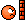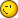# Why Answer From Solve Equation Exit Only One Answer(when It Should Hav

2 replies to this topic

### #1tamm

tamm

Newbie

•• Members
•• 1 posts

• Calculators:

Posted 04 October 2005 - 08:46 AM

when type solve(x^1.55/1500-0.344x+1060=0,x) the answer comes only x=79600 when it should have x=3745 too.what do i have to do to get all answer from the equation.### #2PAP

PAP

Casio Overlord

•• Members
•• 681 posts
• Gender:Male
• Location:Somewhere in Europe.
• Interests:Computer Algebra, Numerical Analysis.

• Calculators:
ClassPad 300 (plus an old Casio model, with only a few Kb ram).

Posted 04 October 2005 - 06:16 PM

when type solve(x^1.55/1500-0.344x+1060=0,x) the answer comes only x=79600 when it should have x=3745 too.what do i have to do to get all answer from the equation.This subject has been discussed some months ago, so I will not repeat it here. In short: you are asking for something much more difficult than you think. Locating and computing all the roots of a function is not an easy task. There is a method to do this, but it needs "heavy" mathematics even to understand how it works. But if you want to solve the problem, use LuaNumAn. It's a Numerical Analysis package written by me. You will need the CPLua Add-In to run it. Go to the CPLua project for details.Welcome to the CPLua world!

### #3PAP

PAP

Casio Overlord

•• Members
•• 681 posts
• Gender:Male
• Location:Somewhere in Europe.
• Interests:Computer Algebra, Numerical Analysis.

• Calculators:
ClassPad 300 (plus an old Casio model, with only a few Kb ram).

Posted 06 October 2005 - 08:57 AM

Here are two screenshots, showing the solution of tamm's problem using LuaNumAn:(the fist screenshot shows the console window after executing a simple CPLua program that uses LuaNumAn, and the second is a graph of the function together with its roots, as computed by the program). The graph is generated by LuaNumAn plotting functions.
The computation time for locating and computing all the roots of tamm's function within the interval [0,100000] is less than one second (you press "Run", and you get the roots almost instantly)#### 0 user(s) are reading this topic

0 members, 0 guests, 0 anonymous users